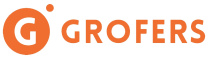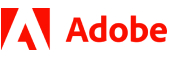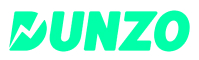New update is available. Click here to update.
Last Updated: 24 Jul, 2021

# Longest Common Prefix

Moderate+13 more companies

## Problem statement#### A prefix of a string can be defined as a substring obtained after removing some or all characters from the end of the string.

##### For Example:
``````Consider ARR = [“coding”, ”codezen”, ”codingninja”, ”coders”]
The longest common prefix among all the given strings is “cod” as it is present as a prefix in all strings. Hence, the answer is “cod”.
``````
##### Input Format:
``````The first line of the input contains a single integer, 'T,’ denoting the number of test cases.

The first line of each test case contains a single integer ‘N’ denoting the number of strings in the array.

The next line contains ‘N’ space-separated strings denoting the elements of the array ‘ARR’.
``````
##### Output Format:
``````For each test case, print a single string corresponding to the longest common prefix.

Print the output of each test case in a separate line.
``````
##### Note:
``````You do not need to print anything. It has already been taken care of. Just implement the given function.
``````
##### Constraints:
``````1 <= T <= 10
1 <= N <= 3000
1 <= |ARR[i]| <=1000

Each string consists of only lowercase letters.

Time limit: 1 sec
``````## Approaches

### 01 ApproachIn this approach, we will iterate through each character of the first string and check if the same character exists in each string or not. We will maintain a variable longestPrefix to store the longest common prefix.

We will traverse idx from 0 to the length of ARR -1.

1. We will iterate index from 1 to N-1 and check if ARR[index][idx] is equal to ARR[idx].
2. If the condition is true for all strings, we can add ARR[idx] in the common prefix string, and we will insert ARR[idx] in longestPrefix. Otherwise, the search is completed for the longest common prefix.

In the end, we will return the variable longestPrefix.

#### Algorithm:

• We will declare a string longestPrefix to store the longest prefix string of all strings.
• We will iterate through the first string of ARR and check each of its characters is present in all other strings or not.
• Iterate idx from 0 to length of ARR - 1 ,do the following:
• Set ch as ARR[idx]. The variable ch stores the character to be searched.
• Set matched as true. The variable stores if it is possible to insert ch into the longestPrefix.
• We will iterate index from 1 to N-1, do the following:
• Check if the length of ARR[index] is less than or equal to the variable idx or ARR[index][idx] is not equal to ch.
• Set matched as false.
• Break this loop.
• If matched is true, we can insert character ch into longestPrefix. Otherwise, break this loop as the longest common prefix is already found.
• Return the string longestPrefix corresponding to the longest prefix string of all the strings in the given array.